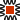### Critical points to consider about Inductance and Capacitance:Everything about Capacitors is totally opposite of an Inductor!Inductors and Capacitors Store Energy in their respective fields.They do not Dissipate (Transform) Energy like Resistance does, like in the form of heat, or radiation.In an antenna, they give it back to the XMTR, rather than Radiating it as Power Transmitted.In this case, the antenna looks like pure resistance, at resonance.Inductors do not like CHANGES in current. (they "React" to those changes, see also "Time Constants" below)Capacitors do not like CHANGES in voltage. (they "React" to those changes, see also "Time Constants" below)In both cases the RATE of CHANGE is critical.This is why the formulas for Inductive Reactance and Capacitive Reactance have Frequency as a primary component.Inductors oppose an increase in current by producing a CEMF against the suplied voltageThey also appear as an open circuit to the change, to the source. (see L&C Comparisons)Capacitors oppose an increase in voltage by drawing current from the source from the supplyThey look like a short circuit to the change, to the source. (see L&C Comparisons)Inductors use the applied energy to store it in an ElectroMagnetic Field, as current begins to flow (increase).Capacitors use the applied energy to store it in an ElectroStatic Field as the voltage charge increases (and current decreases).As the "Rate of Change" increases, as in the frequency that is applied, an inductor will oppose the change in current more and more, therefore appearing as a higher ohms value.Hence the formula XL = 2*(pi)*freq*HenryAs the "Rate of Change" increases, as in the frequency that is applied, a capacitor will draw more and more current, therefore appearing as a lower ohms value.Hence the formula XC = 1/(2*(pi)*frequency*Farads)

(with the reciprical relationship decreasing the Ohms as frequency increases)

Examples:Power supply filter system (Concerns about "Inrush Current")Low-Pass-Filter, High-Pass-Filter, BandPass-Filter, Notch-Filter (Band-Pass-Reject)Oscillator-Tuned-CircuitsTime Constants,

(more on Time Constants)Automotive Spark coil (and capacitor)

{ Windherst & VanDeGraph Systems to charge a home-made capacitor }

Explaining_Reactance.html - ddf – WA7RSO – 09/21/2020